# 1 Introduction

This is a practical user guide to colourvision. Colour vision models allow appraisals of colour patches independently of the human vision. More detailed explanation on colour vision models is provided elsewhere (Kelber, Vorobyev, and Osorio 2003; Endler and Mielke 2005; Osorio and Vorobyev 2008; Kemp et al. 2015; Renoult, Kelber, and Schaefer 2017; Gawryszewski 2018).

Package colourvision has functions to the three most commonly used models by ecologists, behavioural ecologists, and evolutionary biologists (Chittka 1992; Vorobyev and Osorio 1998; Vorobyev et al. 1998; Endler and Mielke 2005), and a generic function to build alternative models based on the same set of general assumptions. These models have been extended to accept any number of photoreceptor types, i.e., the same model may be applied to a dichromatic mammal and a tentatively pentachromatic dipterean. Modelling functions provide a comprehensive output, which may be visualised into publication-ready colour space plots.

# 2 Data handling

This section shows functions applied to colour data before model calculations.

## 2.1spec.denoise()

Applies a smooth.spline for a data frame containing spectrometric data. Useful when raw data from spectrophotometer output are noisy.

spider.smooth<-spec.denoise(spider)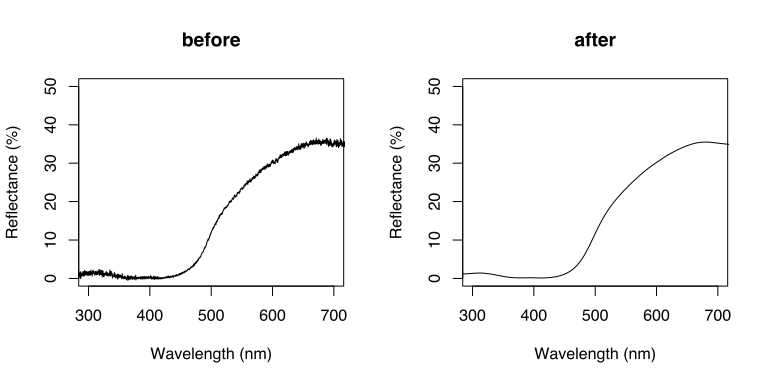Figure 1. Effect of spec.denoise( ) applied to a reflectance curve.

## 2.2photor()

Photoreceptor sensitivity curves are seldom available, but they can be estimated using the wavelength of maximum sensitivity ($$\lambda_{max}$$; Govardovskii et al. 2000). photor generates photoreceptor sensitivity spectra based on $$\lambda_{max}$$ values:

human<-photor(lambda.max = c(420,530,560), lambda = seq(400, 700, 1))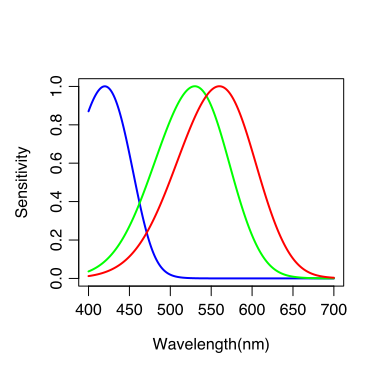Figure 2. Estimated photoreceptor sensitivity curves based on the wavelength of maximum sensitivity using photor( ) function.

## 2.3logistic()

Creates a sigmoid reflectance curve. Useful for simulations using colour vision models (see for instance Gawryszewski 2018).

R<-logistic(x0=500, L=80, k=0.04)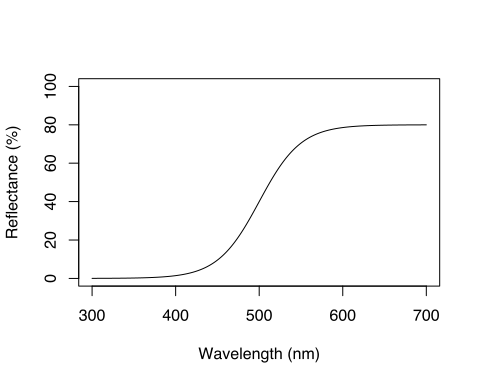Figure 3. Simulated reflectance spectrum using the logistic( ) function.

## 2.4energytoflux()

Photoreceptors respond to photon numbers, not photon energy (Endler 1990). energytoflux converts Irradiance datum from energy units ($$uW \times cm^{-2} \times nm^{-1}$$) to quantum flux units ($$umol \times m^{-2} \times s^{-1}$$).

D65photon<-energytoflux(D65energy)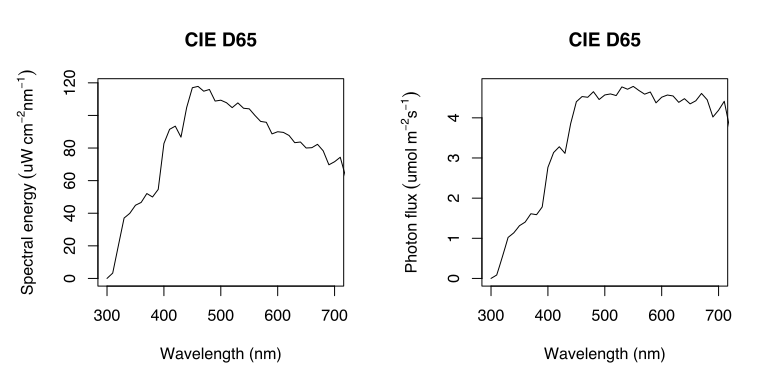Figure 4. CIE D65 in photon energy (left) and quantum flux units (right)

# 3 Colour Vision Models

## 3.1 The Basics

This section serves as a brief introduction to colour vision models, and to introduce internal package functions used to calculate colour vision models. For model specific functions (CTTKmodel, EMmodel, RNLmodel, RNLthres, and GENmodel), please refer to further sections in this manual.

Colour vision models require a minimum of four parameters for calculations: (1) photoreceptor sensitivity curves, (2) background reflectance spectrum, (3) illuminant spectrum, and (4) the stimulus reflectance spectrum (stimulus). Receptor noise limited models also require photoreceptor noise for each photoreceptor type.

Firstly, one needs to estimate photon catches of each photoreceptor type in the retina, which is a function of the stimulus reflectance, photoreceptor sensitivity, and the illuminant spectrum:

$Q_i = \int_{300}^{700} R(\lambda)I(\lambda)C_i(\lambda) d\lambda$ where $$R(\lambda)$$ denote the stimulus reflectance, $$I(\lambda)$$ the illuminant spectrum, and $$C(\lambda)$$ the photoreceptor sensitivity curves. Note that the illuminant spectrum has to be in quantum flux units, not energy units, because photoreceptors respond to photon numbers, not photon energy.

In colourvision quantum catches are represented by function Q. Here, quantum catches of a given stimulus (R) are estimated by each photoreceptor type found in Apis mellifera (bee; Peitsch et al. 1992) under the CIE D65 standard illuminant (D65):

R<-logistic(x=seq(300,700,1), x0=500, L=80, k=0.04)
data("bee")
data("D65")

Qcatch1<-Q(R=R,I=D65,C=bee[c(1,2)],interpolate=TRUE,nm=seq(300,700,1))
Qcatch2<-Q(R=R,I=D65,C=bee[c(1,3)],interpolate=TRUE,nm=seq(300,700,1))
Qcatch3<-Q(R=R,I=D65,C=bee[c(1,4)],interpolate=TRUE,nm=seq(300,700,1))

In general, colour vision models assume that photoreceptors are adapted to the background. This is achieved by calculating quantum catch of each photoreceptor type in relation to the quantum catches based on the background reflectance (also known as the von Kries transformation). $Qb_i = \int_{300}^{700} Rb(\lambda)I(\lambda)C_i(\lambda) d\lambda$

$q_i = \frac{Q_i}{Qb_i}$

where $$Rb$$ is the background reflectance, $$Q_i$$ is the quantum catch from the stimulus reflectance, and $$Qb_i$$ is the quantum catch from the background reflectance.

Relative quantum catches are calculated using function Qr. Here photoreceptors are assumed to be adapted to a reflectance background based on samples collected in the Brazilian savanna (Gawryszewski and Motta 2012):

data("Rb")

Qr1<-Qr(R=R,Rb=Rb, I=D65,C=bee[c(1,2)],interpolate=TRUE,nm=seq(300,700,1))
Qr2<-Qr(R=R,Rb=Rb, I=D65,C=bee[c(1,3)],interpolate=TRUE,nm=seq(300,700,1))
Qr3<-Qr(R=R,Rb=Rb, I=D65,C=bee[c(1,4)],interpolate=TRUE,nm=seq(300,700,1))

The relationship between photoreceptor input and output are assumed to be non-linear. Each colour vision model uses a different transformation function (e.g., log, x/(x+1)), but with the same general result: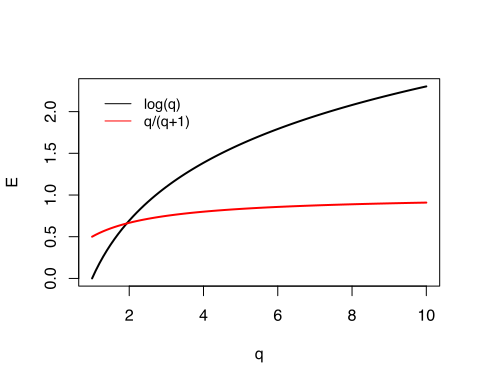Figure 5. Relationship between photoreceptor input and output typically used in colour vision models.

For instance, Chittka (1992) assumes an asymptotic curve with limit = 1: $E_i = \frac{q_i}{q_i+1}$

Photoreceptor outputs are then projected as equidistant vectors into a colour space. Again, each model will present differently arranged vectors, however, this arrangement is arbitrary and has no effect on model predictions. The length of the resultant vector represents the chromaticity distance of the stimulus in relation to the background, and vector coordinates represent the stimulus locus in the animal colour space (colour locus).

For instance, colour_space generates a general colour space based on any number of photoreceptor types, and calculates colour locus coordinates for a given photoreceptor output:

colour_space(n=3, type="length", length=1, edge=NA, q=c(Qr1,Qr2,Qr3))
## $coordinates ## X1 X2 ## 1.458867 4.329547 ## ##$vector_matrix
##            v1         v2 v3
## X1 -0.8660254  0.8660254  0
## X2 -0.5000000 -0.5000000  1

## 3.2 Chittka (1992) Colour Hexagon

Chittka (1992) developed a colour vision model based on trichromatic hymenopteran vision. This model has later been extended to tetrachromatic avian vision (Thery and Casas 2002). In colourvision this model has been further extended to accept any number of photoreceptor types ($$n\geq2$$).

Photoreceptor outputs ($$E_i$$) are calculated by: ${E_i = \frac{q_i}{q_i+1}}$

Then, for trichromatic vision, coordinates in the colour space are found by (Chittka 1992): ${X_1 = \frac{\sqrt{3}}{2}(E_3-E_1)}$ ${X_2 = E_2-\frac{1}{2}(E_1+E_3)}$ For tetrachromatic vision (Thery and Casas 2002): ${X_1 = \frac{\sqrt{3}\sqrt{2}}{3}(E_3-E_4)}$ ${X_2 = E_1-\frac{1}{3}(E_2+E_3+E_4)}$ ${X_3 = \frac{2\sqrt{2}}{3}(\frac{1}{2}(E_3+E_4)-E_2)}$ Then, for a pentachromatic animal (Gawryszewski 2018): ${X_1 = \frac{5}{2\sqrt{2}\sqrt{5}}(E_2-E_1)}$ ${X_2 = \frac{5\sqrt{2}}{2\sqrt{3}\sqrt{5}}(E_3-\frac{E_1+E_2}{2})}$ ${X_3 = \frac{5\sqrt{3}}{4\sqrt{5}}(E_4-\frac{E_1+E_2+E_3}{3})}$ ${X_4 = E_5-\frac{E1+E2+E3+E4}{4}}$

### 3.2.1CTTKmodel()

Chittka (1992) model is represented by function CTTKmodel. This functions needs (1) photoreceptor sensitivities curves, (2) background reflectance spectrum, (3) illuminant spectrum, and (4) stimulus reflectance spectra:

A worked example:

1. Load data files: Apis mellifera photoreceptor sensitivity curves (Peitsch et al. 1992), Background reflectance and the illuminant spectrum:
data("bee")
data("Rb")
data("D65")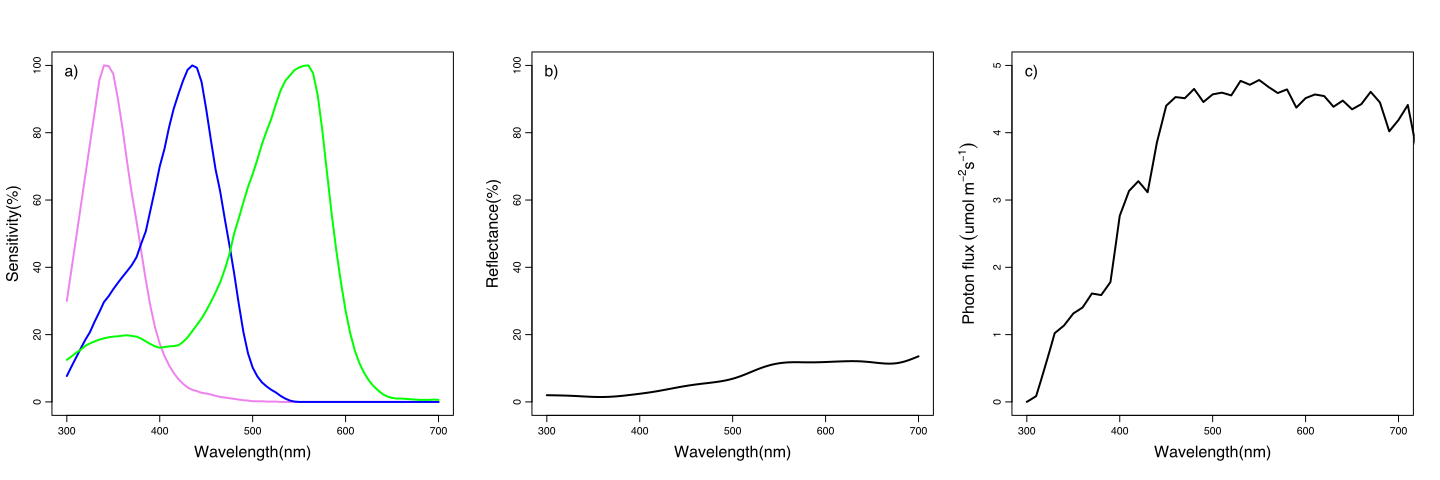Figure 6. Apis mellifera photoreceptor sensitivity curves (a; data from Peitsch et al. 1992), background reflectance spectrum (b; data from Gawryszewski and Motta 2012), and CIE D65 standard illuminant (c).

2. Create simulated reflectance data:

midpoint<-seq(from = 500, to = 600, 10)
W<-seq(300, 700, 1)
R<-data.frame(W)
for (i in 1:length(midpoint)) {
R[,i+1]<-logistic(x = seq(300, 700, 1), x0=midpoint[[i]], L = 70, k=0.04)[,2]+5
}
names(R)[2:ncol(R)]<-midpoint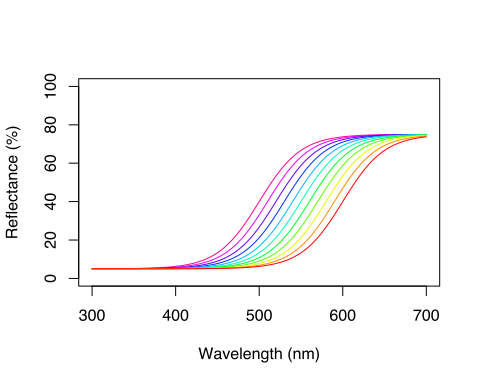Figure 7. Simulated reflectance spectra.

3. Run Chittka (1992) model:

CTTKmodel3<-CTTKmodel(R=R, I=D65, C=bee, Rb=Rb)

Model output provides the relative quantum catches (Qr), photoreceptor outputs (E), colour locus coordinates (X), and the chromaticity distance of stimulus in relation to the background (deltaS).

CTTKmodel3

Table 1. CTTKmodel() output of a trichromatic animal, showing the relative quantum catches (Qr), photoreceptor outputs (E), colour locus coordinates (X), and the chromaticity distance of stimulus in relation to the background (deltaS)
Qr1 Qr2 Qr3 E1 E2 E3 X1 X2 deltaS
500 3.109465 3.304717 5.684952 0.7566593 0.7676967 0.8504103 0.0811907 -0.0358381 0.0887485
510 2.936685 2.759015 5.269557 0.7459792 0.7339729 0.8404991 0.0818566 -0.0592663 0.1010594
520 2.809985 2.336604 4.825336 0.7375318 0.7002941 0.8283361 0.0786388 -0.0826398 0.1140763
530 2.718425 2.018160 4.360565 0.7310689 0.6686723 0.8134525 0.0713462 -0.1035884 0.1257809
540 2.653176 1.783585 3.885885 0.7262656 0.6407511 0.7953288 0.0598105 -0.1200461 0.1341207
550 2.607271 1.614217 3.413825 0.7227822 0.6174763 0.7734391 0.0438702 -0.1306343 0.1378039

## 3.3 Endler & Mielke (2005)

The original model is available for tetrachromatic animals only. In colourvision, the model was extended to any number of photoreceptors (Gawryszewski 2018; see also Pike 2012).

First, relative quantum catches are log-transformed:

$f_i = \ln(q_i)$

where $$q_i$$ is the relative quantum catch of each photoreceptor type. The model uses only relative values, so that photoreceptor outputs ($$E$$) are given by:

$E_i = \frac{f_i}{f_1+f_2+f_3+...+f_n}$

Then, for tetrachromatic vision colour locus coordinates are found by (Endler and Mielke 2005):

$X_1 = \sqrt{\frac{3}{2}}(1-2\frac{E2-E3-E1}{2})$ ${X_2 = \frac{-1+3E_3+E_1}{2\sqrt{2}}}$ ${X_3 = E_1-\frac{1}{4}}$

Tetrachromatic chromaticity diagram (tetrahedron) in Endler and Mielke (2005) has a maximum photoreceptor vector of length = 0.75, which gives a tetrahedron with edge length = $$\sqrt{\frac{3}{2}}$$. The chromaticity coordinates for other colour spaces may preserve either the same vector length or the same edge length.

For instance, for dichromatic vision, coordinate (X1) in the colour space preserving the same vector length is found by:

${X_1 = \frac{3}{4}(E_2-E_1)}$

whereas if the edge length is preserved, $$X_1$$ is found by:

$\frac{1}{2}\sqrt{\frac{3}{2}}(E_2-E_1)$

### 3.3.1EMmodel()

Using the same data as in CTTKmodel() example:

EMmodel3<-EMmodel(type="length",R=R,I=D65,Rb=Rb,C=bee)

Model output provides the relative quantum catches (Qr), photoreceptor outputs (E), colour locus coordinates (X), and the chromaticity distance of stimulus in relation to the background (deltaS).

Table 2. EMmodel() output of a trichromatic animal, showing the relative quantum catches (Qr), photoreceptor outputs (E), colour locus coordinates (X), and the chromaticity distance of stimulus in relation to the background (deltaS)
Qr1 Qr2 Qr3 E1 E2 E3 X1 X2 deltaS
500 3.109465 3.304717 5.684952 0.2788976 0.2938695 0.4272328 0.0097246 0.1056369 0.1060836
510 2.936685 2.759015 5.269557 0.2869612 0.2703373 0.4427015 -0.0107975 0.1230392 0.1235120
520 2.809985 2.336604 4.825336 0.2989732 0.2455897 0.4554371 -0.0346736 0.1373667 0.1416752
530 2.718425 2.018160 4.360565 0.3149930 0.2211722 0.4638348 -0.0609384 0.1468141 0.1589588
540 2.653176 1.783585 3.885885 0.3351122 0.1987220 0.4661658 -0.0885880 0.1494365 0.1737214
550 2.607271 1.614217 3.413825 0.3595905 0.1796819 0.4607275 -0.1168540 0.1433185 0.1849191

## 3.4 Receptor Noise Limited Models (Vorobyev & Osorio 1998; Vorobyev et al. 1998)

Receptor Noise Limited Model assumes that chromatic discrimination is limited by noise at the photoreceptors (Vorobyev and Osorio 1998; Vorobyev et al. 1998). Model calculation follows similar steps as in Chittka (1992) and Endler and Mielke (2005), but has an additional step, namely, calculation of noise at the resultant vector, based on noise of each photoreceptor type.

Photoreceptor noise is seldom measured directly. In lack of direct measurement, receptor noise ($${e_i}$$) can be estimated by the relative abundance of photoreceptor types in the retina, and a measurement of a single photoreceptor noise-to-signal ratio (Vorobyev and Osorio 1998; Vorobyev et al. 1998): ${e_i=\frac{\nu}{\sqrt{\eta _i}}}$ where $${\nu}$$ is the noise-to-signal ratio of a single photoreceptor, and $${\eta}$$ is the relative abundance of photoreceptor $$i$$ in the retina.

Vorobyev and Osorio (1998) aimed to predict colour thresholds. Close to the threshold, the relationship between photoreceptor input and output is nearly linear, so that $$E_i=q_i$$. However, for comparison between two colours that are not perceptually near the threshold, one must use a non-linear relationship between photoreceptor input and output (Vorobyev et al. 1998): $$E_i=ln(q_i)$$.

Then, $$\Delta$$S is calculated by (eq. A7 in Vorobyev et al. 1998):

$(\Delta{S})^2 = V \Delta\vec{p} \bullet (V R V^T)^{-1} V \Delta\vec{p}$ where $$V$$ is a matrix of column vectors, $$\bullet$$ denotes the inner product, $$T$$ denotes the transpose, $$\Delta\vec{p}$$ is a vector which components represent differences between $$E$$-values, and $$R$$ is a covariance matrix of photoreceptor values. Photoreceptors values are not correlated, therefore $$R$$ is a diagonal matrix in which diagonal elements are the photoreceptor variances ($$e_i^2$$):

$R = \left[\array{ e_1^2 & 0 & 0 &\\ 0 & e_2^2 & 0 &\cdots &\\ 0 & 0 & e_3^2&\\ & \vdots & & \ddots &}\right]$

The receptor noise limited model was originally developed to calculate $$\Delta$$S between two reflectance curves directly, without finding colour locus coordinates (see eqs 3-5 in Vorobyev and Osorio 1998). Nonetheless, for visualisation purposes it is useful to project colour vision model results into chromaticity diagrams. This can be done by (Gawryszewski 2018; see also Hempel de Ibarra, Giurfa, and Vorobyev 2001; and Renoult, Kelber, and Schaefer 2017 for alternative formulae):

$\vec{s} = \sqrt{(V R V^T)^{-1}} V \vec{p}$

where $$\vec{s}$$ is a vector which components represent stimulus colour locus coordinates, $$\vec{p}$$ is a vector which components represent stimulus $$E$$-values, and other elements are the same as in the preceding formula.

### 3.4.1RNLmodel()

Using the same data as in CTTKmodel() example:

RNLmodel3<-RNLmodel(model="log",photo=3,R1=R,Rb=Rb,I=D65,C=bee,
noise=TRUE,e=c(0.13,0.06,0.11))

Model above calculates $$\Delta$$S values based on noise measured at Apis mellifera photoreceptors (e=c(0.13,0.06,0.11)). Alternatively, noise might have been calculated based on photoreceptor relative abundances:

RNLmodel3.1<-RNLmodel(model="log", photo=3, R1=R, Rb=Rb, I=D65, C=bee,
noise=FALSE, n=c(1,2,1), v=0.1)

Furthermore, users might add another reflectance stimulus (R2) to be compared against the first stimulus (R1):

R2<-logistic(x = seq(300, 700, 1), x0=512, L = 70, k=0.01)
RNLmodel3.2<-RNLmodel(model="log", photo=3, R1=R, R2=R2, Rb=Rb, I=D65, C=bee, noise=FALSE, n=c(1,2,1), v=0.1)

Model output provides photoreceptor noise (e), the relative quantum catches (Qr), photoreceptor outputs (E), colour locus coordinates (X), and the chromaticity distance (deltaS) of the first stimulus in relation to the second stimulus (against the background when R1=Rb).

head(RNLmodel3.2)
##      e1         e2  e3   Qr1_R1   Qr2_R1   Qr3_R1   Qr1_R2   Qr2_R2
## 500 0.1 0.07071068 0.1 3.109465 3.304717 5.684952 6.893501 5.511065
## 510 0.1 0.07071068 0.1 2.936685 2.759015 5.269557 6.893501 5.511065
## 520 0.1 0.07071068 0.1 2.809985 2.336604 4.825336 6.893501 5.511065
## 530 0.1 0.07071068 0.1 2.718425 2.018160 4.360565 6.893501 5.511065
## 540 0.1 0.07071068 0.1 2.653177 1.783585 3.885885 6.893501 5.511065
## 550 0.1 0.07071068 0.1 2.607272 1.614217 3.413825 6.893501 5.511065
##      Qr3_R2     E1_R1     E2_R1    E3_R1    E1_R2    E2_R2    E3_R2
## 500 4.10912 1.1344507 1.1953509 1.737823 1.930579 1.706758 1.413209
## 510 4.10912 1.0772815 1.0148737 1.661946 1.930579 1.706758 1.413209
## 520 4.10912 1.0331792 0.8486987 1.573880 1.930579 1.706758 1.413209
## 530 4.10912 1.0000527 0.7021864 1.472602 1.930579 1.706758 1.413209
## 540 4.10912 0.9757576 0.5786256 1.357351 1.930579 1.706758 1.413209
## 550 4.10912 0.9583043 0.4788501 1.227833 1.930579 1.706758 1.413209
##           X1_R1    X2_R1     X1_R2     X2_R2   deltaS
## 500  0.04797592 4.898812 -1.527249 -3.342552 8.390555
## 510 -1.00503765 5.354027 -1.527249 -3.342552 8.712244
## 520 -2.02728486 5.585260 -1.527249 -3.342552 8.941805
## 530 -2.95508194 5.564463 -1.527249 -3.342552 9.020733
## 540 -3.74247569 5.276414 -1.527249 -3.342552 8.899090
## 550 -4.36636037 4.721614 -1.527249 -3.342552 8.549347

### 3.4.2RNLthres()

Vorobyev and Osorio (1998) aimed to predict discrimination threshold of monochromatic stimuli. By definition, thresholds are found when $$\Delta{S} = 1$$, therefore (Vorobyev and Osorio 1998):

$(1)^2 = V \Delta\vec{p} \bullet (V R V^T)^{-1} V \Delta\vec{p}$ RNLthres() calculates thresholds of monochromatic light for a given background, illuminant, photoreceptor sensitivities and photoreceptor noise.

Worked example:

1. Bee photoreceptors normalized 1.
C<-bee
C[,2]<-C[,2]/max(C[,2])
C[,3]<-C[,3]/max(C[,3])
C[,4]<-C[,4]/max(C[,4])
1. Grey background:
Rb.grey <- data.frame(300:700, rep(0.1, length(300:700)))
1. Model calculation:
thres<-RNLthres(photo=3, Rb=Rb, I=D65, C=C,
noise=TRUE, e = c(0.13, 0.06, 0.12))

The output is a data.frame with threshold values (T) and log of the sensitivity values (S), per wavelength (nm). Sensitivity is simply the inverse of threshold ($$S = \frac{1}{T}$$)

Table 3. RNLthres() output, showing wavelength (nm), threshold values (T) and log of the sensitivity values (S).
nm T S
300 83.79800 -4.428409
301 78.86544 -4.367743
302 74.48122 -4.310547
303 70.55872 -4.256445
304 67.02866 -4.205120
305 63.83496 -4.156301

## 3.5 Generic model

A generic function (GENmodel) is provided that allows calculation of alternative models based on the same assumptions of other models. Note, however, that colour locus coordinates may differ because positions of vectors used in GENmodel are not necessarily the same as in each model specific formula. Also, caution should be taken because models generated by GENmodel are not supported by experimental data.

### 3.5.1GENmodel()

Worked examples:

In this case, GENmodel is applying the same transformation (func=function(x){x/(1+x)}), and the colour space has the same maximum vector length (length=1) as in CTTKmodel:

CTTKmodel3<-CTTKmodel(photo=3,R=R,Rb=Rb,I=D65,C=bee)

ANY.CTTKmodel3any<-GENmodel(photo=3, type="length", length=1,R=R,Rb=Rb,I=D65,C=bee, vonKries = TRUE, func=function(x){x/(1+x)}, unity=FALSE,recep.noise=FALSE)

Note, however, that although Qr, E and deltaS values are exactly the same, colour locus coordinates (X) differ between models (Tables 1 and Table 4). This happens because GENmodel uses a different arrangement of vectors than Chittka (1992). The arrangement of photoreceptors output vectors is arbitrary, has no biological meaning, and no effect on model predictions.

ANY.CTTKmodel3any

Table 4. GENmodel( ) output, with model parameters based on the same set of assumptions as in Chittka (1992)
Qr1 Qr2 Qr3 E1 E2 E3 X1 X2 deltaS
500 3.109465 3.304717 5.684952 0.7566593 0.7676967 0.8504103 0.0095586 0.0882323 0.0887485
510 2.936685 2.759015 5.269557 0.7459792 0.7339729 0.8404991 -0.0103978 0.1005231 0.1010594
520 2.809985 2.336604 4.825336 0.7375318 0.7002941 0.8283361 -0.0322488 0.1094231 0.1140763
530 2.718425 2.018160 4.360565 0.7310689 0.6686723 0.8134525 -0.0540370 0.1135818 0.1257809
540 2.653176 1.783585 3.885885 0.7262656 0.6407511 0.7953288 -0.0740578 0.1118204 0.1341207
550 2.607271 1.614217 3.413825 0.7227822 0.6174763 0.7734391 -0.0911975 0.1033099 0.1378039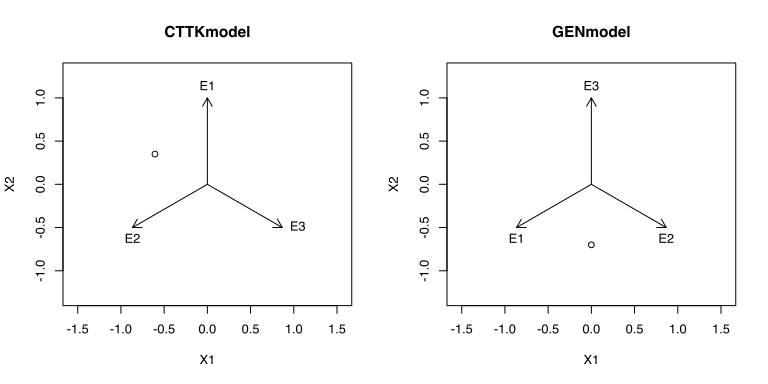Figure 8. Photoreceptors outputs represented as vectors in a colour space. Each colour vision model uses differently arranged vectors. As long as pairwise angles between vectors are identical, vector arrangements have no biological significance.

Alternatively, users may choose to change some aspect of models. In the example below, a model based on receptor noise is calculated, but with a different photoreceptor input-output transformation:

RNLmodel3<-RNLmodel(model="log",photo=3,R1=R,Rb=Rb,I=D65,C=bee,
noise=TRUE,e=c(0.13,0.06,0.11))

ANY.RNLmodel3any<-GENmodel(photo=3, type="length", length=1,R=R,Rb=Rb,I=D65,C=bee, vonKries = TRUE, func=function(x){x/(1+x)}, unity=FALSE,recep.noise=TRUE, noise.given=TRUE,e=c(0.13,0.06,0.11))

Note that in this case several parameters differ between models:

head(RNLmodel3[,c("E1_R1","E2_R1","E3_R1","X1_R1","X2_R1","deltaS")])

Table 5. RNLmodel( ) results, showing photoreceptor outputs (Ei_R1), colour locus coordinates (Xi_R1), and the chromaticity distance to the background (deltaS).
E1_R1 E2_R1 E3_R1 X1_R1 X2_R1 deltaS
500 1.1344507 1.1953509 1.737823 -0.4156057 4.507320 4.526440
510 1.0772815 1.0148737 1.661946 -1.3869616 5.012078 5.200441
520 1.0331792 0.8486987 1.573880 -2.3102433 5.308077 5.789034
530 1.0000527 0.7021864 1.472602 -3.1266568 5.364311 6.209011
540 0.9757576 0.5786256 1.357351 -3.7942469 5.163031 6.407277
550 0.9583043 0.4788501 1.227833 -4.2926799 4.702820 6.367387

head(ANY.RNLmodel3any[,c("E1","E2","E3","X1","X2","deltaS")])

Table 6. GENmodel( ) based on receptor noise but with a different transformation between photoreceptor input and output. Model results showing photoreceptor outputs (Ei_R1), colour locus coordinates (Xi_R1), and the chromaticity distance to the background (deltaS).
E1 E2 E3 X1 X2 deltaS
500 0.7566593 0.7676967 0.8504103 -0.0518106 0.6919809 0.6939178
510 0.7459792 0.7339729 0.8404991 -0.2397641 0.8204547 0.8547706
520 0.7375318 0.7002941 0.8283361 -0.4386927 0.9246375 1.0234285
530 0.7310689 0.6686723 0.8134525 -0.6299436 0.9907664 1.1740729
540 0.7262656 0.6407511 0.7953288 -0.7972665 1.0068354 1.2842708
550 0.7227822 0.6174763 0.7734391 -0.9299592 0.9645294 1.3398287

## 3.6 Chromaticity distances ($$\Delta$$S)

Chromaticity distances ($$\Delta$$S) are the Euclidean distances between points in the animal colour space. It is frequently assumed that there is a positive and linear relationship between $$\Delta$$S values and the probability of discrimination between two colours (although this is not necessarily the case, see Garcia, Spaethe, and Dyer 2017).

In CTTKmodel, EMmodel, and GENmodel model outputs, deltaS values represent the distance between the stimulus and the background. In RNLmodel output, deltaS represents the distance between R1 and R2, or between R1 and the background when R1=Rb.

However, one may want to compute all pairwise chromaticity distance between all stimuli. This is done by the deltaS function.

### 3.6.1deltaS

deltaS function will calculate a matrix with all possible pairwise comparison between stimulus reflectance spectra.

dS<-deltaS(CTTKmodel3)
dS
##            500        510        520        530        540        550
## 500 0.00000000 0.02343764 0.04687125 0.06846175 0.08687983 0.10187808
## 510 0.02343764 0.00000000 0.02359401 0.04555125 0.06465465 0.08084780
## 520 0.04687125 0.02359401 0.00000000 0.02218159 0.04187766 0.05926490
## 530 0.06846175 0.04555125 0.02218159 0.00000000 0.02009806 0.03855407
## 540 0.08687983 0.06465465 0.04187766 0.02009806 0.00000000 0.01913640
## 550 0.10187808 0.08084780 0.05926490 0.03855407 0.01913640 0.00000000
## 560 0.11458179 0.09549340 0.07595567 0.05719313 0.03927072 0.02087652
## 570 0.12734136 0.11108201 0.09449266 0.07846243 0.06262808 0.04545165
## 580 0.14297463 0.13025419 0.11717462 0.10419581 0.09058492 0.07473277
## 590 0.16350785 0.15451839 0.14492289 0.13478997 0.12316794 0.10850323
## 600 0.18918330 0.18357103 0.17697890 0.16919563 0.15917711 0.14547444
##            560        570        580        590        600
## 500 0.11458179 0.12734136 0.14297463 0.16350785 0.18918330
## 510 0.09549340 0.11108201 0.13025419 0.15451839 0.18357103
## 520 0.07595567 0.09449266 0.11717462 0.14492289 0.17697890
## 530 0.05719313 0.07846243 0.10419581 0.13478997 0.16919563
## 540 0.03927072 0.06262808 0.09058492 0.12316794 0.15917711
## 550 0.02087652 0.04545165 0.07473277 0.10850323 0.14547444
## 560 0.00000000 0.02503866 0.05494735 0.08932603 0.12681114
## 570 0.02503866 0.00000000 0.03011585 0.06475593 0.10248593
## 580 0.05494735 0.03011585 0.00000000 0.03472163 0.07255244
## 590 0.08932603 0.06475593 0.03472163 0.00000000 0.03786149
## 600 0.12681114 0.10248593 0.07255244 0.03786149 0.00000000

It may be useful to visualise deltaS output graphically using the ‘corrplot’ package:

library(corrplot)
## corrplot 0.84 loaded
corrplot(corr=dS, is.corr=FALSE)